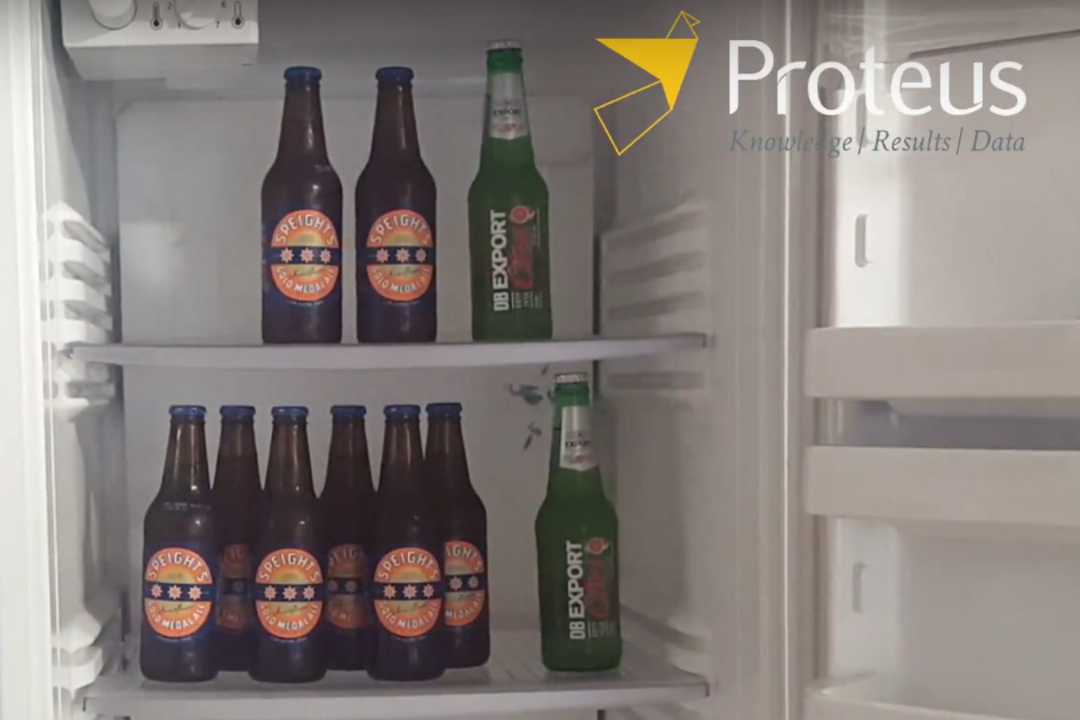In this month's video tip Darryl uses the contents of his beer fridge to explain the relationship between probabilities, odds and odds ratios.

Here's a quick summary. Let Sj=number of Speight's beers and Cj=number of citrus beers on shelf j in the beer fridge. The probability of selecting a Speight's from shelf j, Pj, is therefore:

Pj = Sj/(Sj+Cj),

which makes the probability of selecting a citrus beer 1-Pj=Cj/(Sj+Cj).

The odds compares the probabilities of two outcomes, indicating how much greater the probability of the focal outcome is, and can be calculated as:

Oj = Sj/Cj.

An odds ratio then compares to sets of odds. That is:

OR = O2/O1

Watch the video for more details and further explanations.